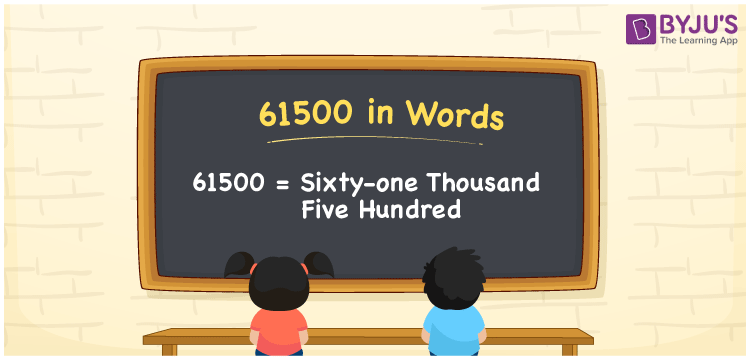# 61500 in Words

61500 in words can be written as Sixty-one thousand five hundred. The fundamental concepts in Mathematics like counting or count can be learnt efficiently here. If you buy a scooter for Rs. 61500, then you can say that “I bought a scooter for Sixty-one thousand five hundred Rupees”. To write numbers in words, the English alphabet is used. The numbers in words concept is explained here in a simple way to improve the conceptual knowledge of students. The 61500 can be read as “Sixty-one thousand five hundred” in English.

 61500 in words Sixty-one thousand five hundred Sixty-one thousand five hundred in Numbers 61500

## 61500 in English Words## How to Write 61500 in Words?

Students can learn about the expanded form and the place value chart of 61500. Five digits are present in the number 61500. With the help of the place value chart given below, students will be able to understand the concepts with ease.

 Ten Thousands Thousands Hundreds Tens Ones 6 1 5 0 0

61500 can be written in expanded form as:

6 x Ten Thousand + 1 x Thousand + 5 × Hundred + 0 × Ten + 0 × One

= 6 x 10000 + 1 x 1000 + 5 × 100 + 0 × 10 + 0 × 1

= 60000 + 1000 + 500

= 61500

= Sixty-one thousand five hundred

Hence, 61500 in words is written as Sixty-one thousand five hundred.

61500 is a natural number that precedes 61501 and succeeds 61499.

61500 in words – Sixty-one thousand five hundred

Is 61500 an odd number? – No

Is 61500 an even number? – Yes

Is 61500 a perfect square number? – No

Is 61500 a perfect cube number? – No

Is 61500 a prime number? – No

Is 61500 a composite number? – Yes

## Frequently Asked Questions on 61500 in Words

Q1

### How to write 61500 in words?

61500 can be written in words as “Sixty-one thousand five hundred”.
Q2

### How to write Sixty-one thousand five hundred in numbers?

Sixty-one thousand five hundred in numbers can be written as 61500.
Q3

### Is 61500 an odd or even number?

61500 is an even number as it is completely divisible by 2.
61500/2 = 30750